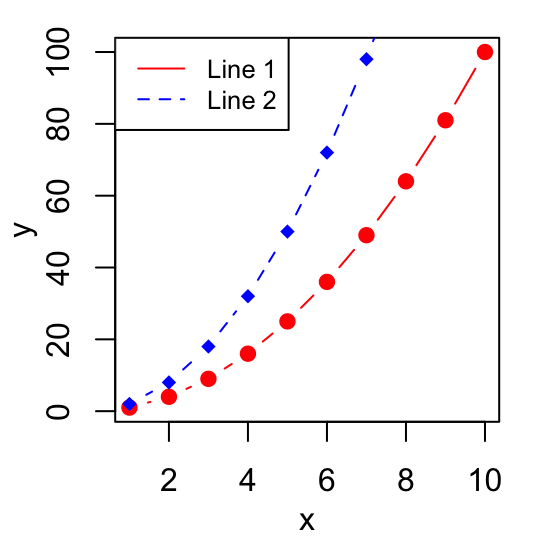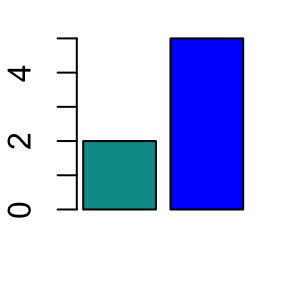# Graphical parameters

This article provides a quick start guide to change and customize R graphical parameters, including:

• adding titles, legends, texts, axis and straight lines
• changing axis scales, plotting symbols, line types and colors

For each of these graphical parameters, you will learn the simplified format of the R functions to use and some examples.

How this chapter is organized?

• Change main title and axis labels
• title colors
• The font style for titles
• Change the font size
• Use the title() function
• Customize the titles using par() function.

Plot titles can be specified either directly to the plotting functions during the plot creation or by using the title() function (to add titles on an existing plot).

``````# Add titles
barplot(c(2,5), main="Main title",
xlab="X axis title",
ylab="Y axis title",
sub="Sub-title",
col.main="red", col.lab="blue", col.sub="black")
# Increase the size of titles
barplot(c(2,5), main="Main title",
xlab="X axis title",
ylab="Y axis title",
sub="Sub-title",
cex.main=2, cex.lab=1.7, cex.sub=1.2)``````How this chapter is organized?

• R legend function
• Title, text font and background color of the legend box
• Border of the legend box
• Specify legend position by keywords

The legend() function can be used. A simplified format is :

``legend(x, y=NULL, legend, col)``

• x and y : the co-ordinates to be used for the legend. Keywords can also be used for x : “bottomright”, “bottom”, “bottomleft”, “left”, “topleft”, “top”, “topright”, “right” and “center”.
• legend : the text of the legend
• col : colors of lines and points beside the text for legends

``````# Generate some data
x<-1:10; y1=x*x; y2=2*y1
# First line plot
plot(x, y1, type="b", pch=19, col="red", xlab="x", ylab="y")
lines(x, y2, pch=18, col="blue", type="b", lty=2)
legend("topleft", legend=c("Line 1", "Line 2"),
col=c("red", "blue"), lty=1:2, cex=0.8)``````How this chapter is organized?

• Add texts within the graph
• Add text in the margins of the graph
• Add mathematical annotation to a plot

To add a text to a plot in R, the text() function [to draw a text inside the plotting area] and mtext()[to put a text in one of the four margins of the plot] function can be used.

A simplified format for text() is :

``text(x, y, labels)``

• x and y are the coordinates of the texts
• labels : vector of texts to be drawn

``````plot(cars[1:10,], pch=19)
text(cars[1:10,],  row.names(cars[1:10,]),
cex=0.65, pos=1,col="red") ``````How this chapter is organized?

The R function abline() can be used to add straight lines (vertical, horizontal or regression lines) to a graph.

A simplified format is :

``abline(a=NULL, b=NULL, h=NULL, v=NULL, ...)``

• a, b : single values specifying the intercept and the slope of the line
• h : the y-value(s) for horizontal line(s)
• v : the x-value(s) for vertical line(s)

``````# Add horizontal and vertical lines
#++++++++++++++++++++++++++++++++++
plot(cars, pch=19)
abline(v=15, col="blue") # Add vertical line
# Add horizontal line, change line color, size and type
abline(h=60, col="red", lty=2, lwd=3)
# Fit regression line
#++++++++++++++++++++++++++++++++++
require(stats)
reg<-lm(dist ~ speed, data = cars)
coeff=coefficients(reg)
# equation of the regression line :
eq = paste0("y = ", round(coeff,1), "*x ", round(coeff,1))
plot(cars, main=eq, pch=18)
abline(reg, col="blue", lwd=2)``````# Add an axis to a plot

axis() function can be used.

A simplified format is :

``axis(side, at=NULL, labels=TRUE)``

• side : the side of the graph the axis is to be drawn on; Possible values are 1(below), 2(left), 3(above) and 4(right).
• at: the points at which tick-marks are to be drawn.
• labels: vector of texts for the labels of tick-marks.

``````x<-1:4; y=x*x
plot(x, y, pch=18, col="red", type="b",
frame=FALSE, xaxt="n") # Remove x axis
axis(1, 1:4, LETTERS[1:4], col.axis="blue")
axis(3, col = "darkgreen", lty = 2, lwd = 0.5)
axis(4, col = "violet", col.axis = "dark violet", lwd = 2)``````Read more —> Add an axis to a plot with R software.

# Change axis scale : minimum, maximum and log scale

xlim and ylim arguments can be used to change the limits for x and y axis. Format : xlim = c(min, max); ylim = c(min, max).

log transformation can be performed using the parameters : log=“x”, log=“y” or log=“xy”.

``````x<-1:10; y=x*x
plot(x, y) # Simple graph
plot(x, y, xlim=c(1,15), ylim=c(1,150))# Enlarge the scale
plot(x, y, log="y")# Log scale``````# Customize tick mark labels

• Color, font style and font size of tick mark labels :
• Orientation of tick mark labels
• Hide tick marks
• Change the string rotation of tick mark labels
• Use the par() function
``````x<-1:10; y<-x*x
# Simple graph
plot(x, y)
# Custom plot : blue text, italic-bold, magnification
plot(x,y, col.axis="blue", font.axis=4, cex.axis=1.5)``````Read more —> Customize tick mark labels.

# Change plotting symbols

The following points symbols can be used in R :Point symbols can be changed using the argument pch.

``````x<-c(2.2, 3, 3.8, 4.5, 7, 8.5, 6.7, 5.5)
y<-c(4, 5.5, 4.5, 9, 11, 15.2, 13.3, 10.5)
# Change plotting symbol using pch
plot(x, y, pch = 19, col="blue")
plot(x, y, pch = 18, col="red")
plot(x, y, pch = 24, cex=2, col="blue", bg="red", lwd=2)``````# Change line types

The following line types are available in R :Line types can be changed using the graphical parameter lty.

``````x=1:10; y=x*x
plot(x, y, type="l") # Solid line (by default)
plot(x, y, type="l", lty="dashed")# Use dashed line type
plot(x, y, type="l", lty="dashed", lwd=3)# Change line width``````Read more —> Line types in R : lty.

# Change colors

• Built-in color names in R
• Specifying colors by hexadecimal code
• Using RColorBrewer palettes
• Use Wes Anderson color palettes
• Create a vector of n contiguous colors

Colors can be specified by names (e.g col=red) or with hexadecimal code (e.gcol = “#FFCC00”).

``````# use color names
barplot(c(2,5), col=c("blue", "red"))
barplot(c(2,5), col=c("#009999", "#0000FF"))``````(Source: http://www.visibone.com)

RColorBrewer package can also be used to create a nice looking color palettes. Read our article : Colors in R.

Read more —> Colors in R.

# Infos

This analysis has been performed using R statistical software (ver. 3.2.4).